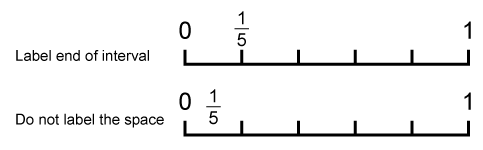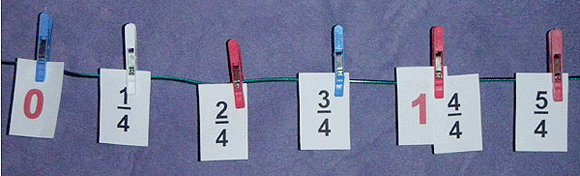Home > Fractions > Good teaching > Fraction sense > Fractions as numbers > Sequencing and counting

# Sequencing and counting

Students peg fraction cards (e.g. $$\frac{1}{5},\frac{2}{5},\frac{3}{5},\frac{4}{5},\frac{5}{5}$$) onto a rope marked with 0 at one end and 1 at the other, adjusting the spacing as needed to create equal intervals. Spacing can be checked by folding the rope.

Focus attention on the distance between 0 and 1 being the whole, and $$\frac{1}{5}$$ being the unit of measure.

So $$\frac{1}{5}$$ is one-fifth of the distance from 0 toward 1.

Highlight the fact that $$\frac{5}{5}$$ is the same as 1.

Ask students to count forwards and backwards by fractions. This reinforces the idea that fractions are indeed numbers, each with their own position on a number line.Correctly labelling number lines.

Change the labelling on the rope number line to extend from 0 to 2 or beyond, and ask students to locate fraction cards such as $$\frac{7}{5}$$ or $$1\frac{2}{5}$$.

Realising that improper fractions and mixed numbers extend fractions beyond 1 is an important part of understanding fractions as numbers.Quarters pegged on a rope.